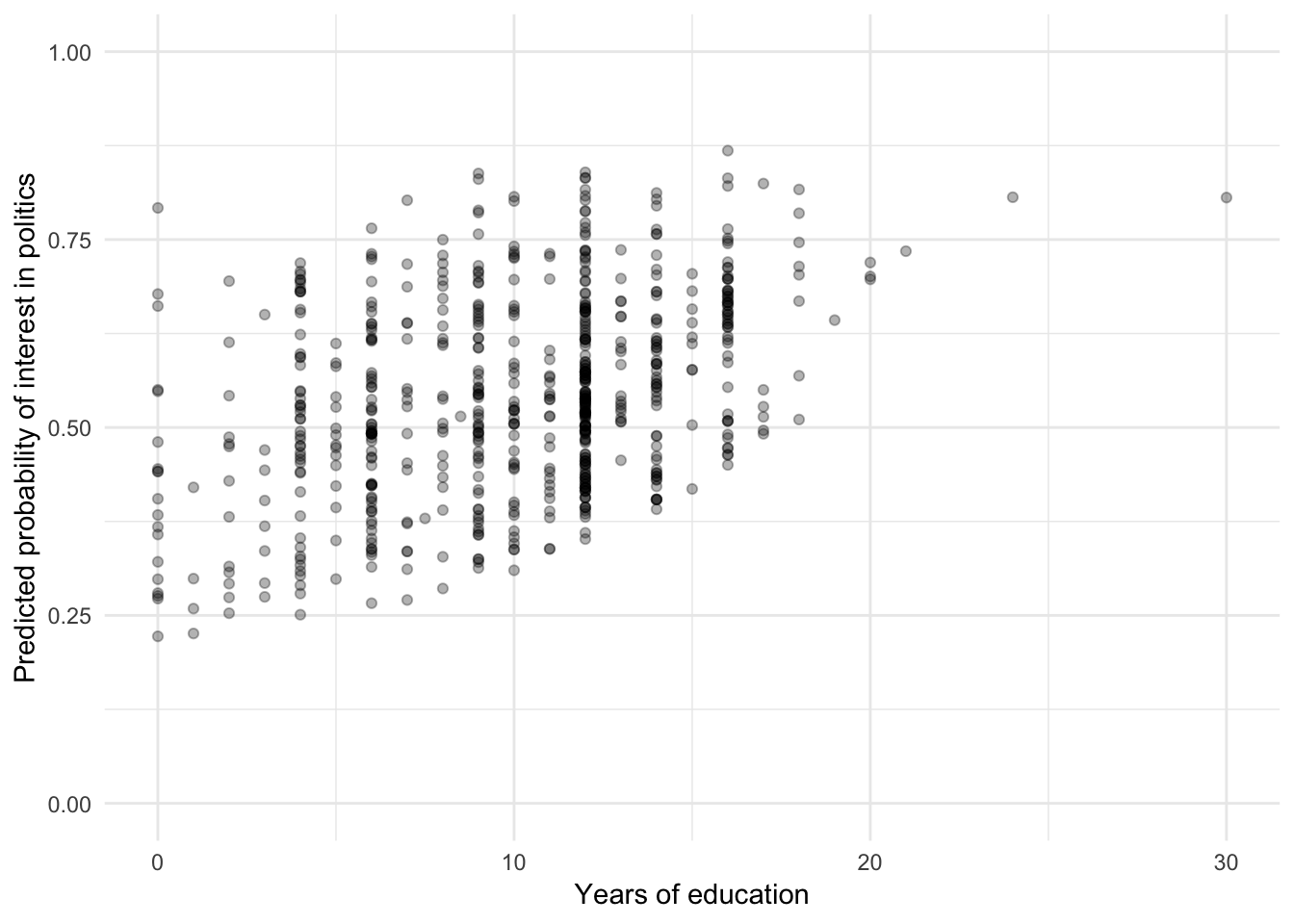library(rio)
library(dplyr)
## Warning: package 'dplyr' was built under R version 3.5.2
##
## Attaching package: 'dplyr'
## The following objects are masked from 'package:stats':
##
##     filter, lag
## The following objects are masked from 'package:base':
##
##     intersect, setdiff, setequal, union
library(stargazer)
##
##  Hlavac, Marek (2018). stargazer: Well-Formatted Regression and Summary Statistics Tables.
##  R package version 5.2.2. https://CRAN.R-project.org/package=stargazer
library(pander)
library(ggplot2)
library(hmeasure)

1 Introduction

In this lab we will look at estimating and evaluating logistic regression models. While the underlying estimation methodology is very different, the implementation in R is quite similar to that of the linear model.

We will make use of the Asiabarometer data available on the teaching data page. There you will also find a brief description of all variables.

When R opens this file, some categorical variables are not properly encoded as factors. This code fixes that:

fixFactor <- function(x) {
labels <- names(attr(x, "labels"))
names(labels) <- unname(attr(x, "labels"))
factor(labels[x])
}

asiabaro\$country <- fixFactor(asiabaro\$country)

2 Performing logistic regression

Here we evaluate which factors affect whether someone is at least somewhat interest in politics:

asiabaro <- asiabaro %>% mutate(somewhatInterested = as.integer(polinterest >= 2))

stargazer(
m0 <- glm(somewhatInterested ~ age,
data = asiabaro,
m1 <- glm(somewhatInterested ~ age + female + urban + education,
data = asiabaro,
type = "html")
 Dependent variable: somewhatInterested (1) (2) age 0.003 0.018*** (0.005) (0.006) female -0.549*** (0.164) urban -0.923*** (0.203) education 0.094*** (0.023) Constant -0.027 -0.629 (0.235) (0.406) Observations 686 665 Log Likelihood -473.843 -434.656 Akaike Inf. Crit. 951.685 879.312 Note: p<0.1; p<0.05; p<0.01

3 Prediction

To obtain predicted probabilities, for example for a plot or for a table, we can use the predict() function. When we set the type parameter to “response”, we get probabilities.

yhat <- predict(m1, type = "response")

We could for example use this to plot predicted probabilities against one of the independent variables:

ggplot(m1\$model, aes(x = education, y = yhat)) +
geom_point(alpha = .3) +
theme_minimal() +
labs(x = "Years of education",
y = "Predicted probability of interest in politics") +
lims(y = c(0,1))Note that we use m1\$model as the data set, which is the data set used in the estimation of model m1, such that all missing data is removed. Otherwise the number of predicted probabilities would not match the number of observations on education.

Furthermore, we fix the scale of the y-axis to 0-1, to avoid suggesting large effects when the actual predicted probabilities vary little.

To predict for a specific kind of individual, we need to create a temporary data frame:

df <- data.frame(
age = 40,
female = 1,
education = 12,
urban = 1
)
predict(m1, newdata = df, type = "response")
##         1
## 0.4375449

We thus find that for a 40 year old female with 12 years of education, living in an urban environment, the predicted probability of at least some interest in politics is 0.44.

We can also do this for a larger temporary data set, for example to produce a plot of predicted probabilities:

df <- expand.grid(
age = 18:90,
education = 12,
female = 0:1,
urban = 0
)
df\$predY <- predict(m1, newdata = df, type = "response")

Let’s have a quick look at this data (showing only the first few observations):

age education female urban predY
18 12 0 0 0.6951
19 12 0 0 0.6989
20 12 0 0 0.7027
21 12 0 0 0.7065
22 12 0 0 0.7102
23 12 0 0 0.7139

We can then use this to plot:

df <- df %>%
mutate(gender = recode(female, `0` = "Male", `1` = "Female"))
ggplot(df, aes(x = age, y = predY, col = gender)) +
geom_line(lwd = 2) +
theme_minimal() +
lims(y = c(0,1)) +
labs(x = "Age",
y = "Predicted probability of interest in politics",
col = "Gender")